# Identify the odd one out using counting methods.

In this worksheet, students will identify an image that does not fit in with the rest of the group. The focus will be on counting, which could involve counting sides, shapes or lines.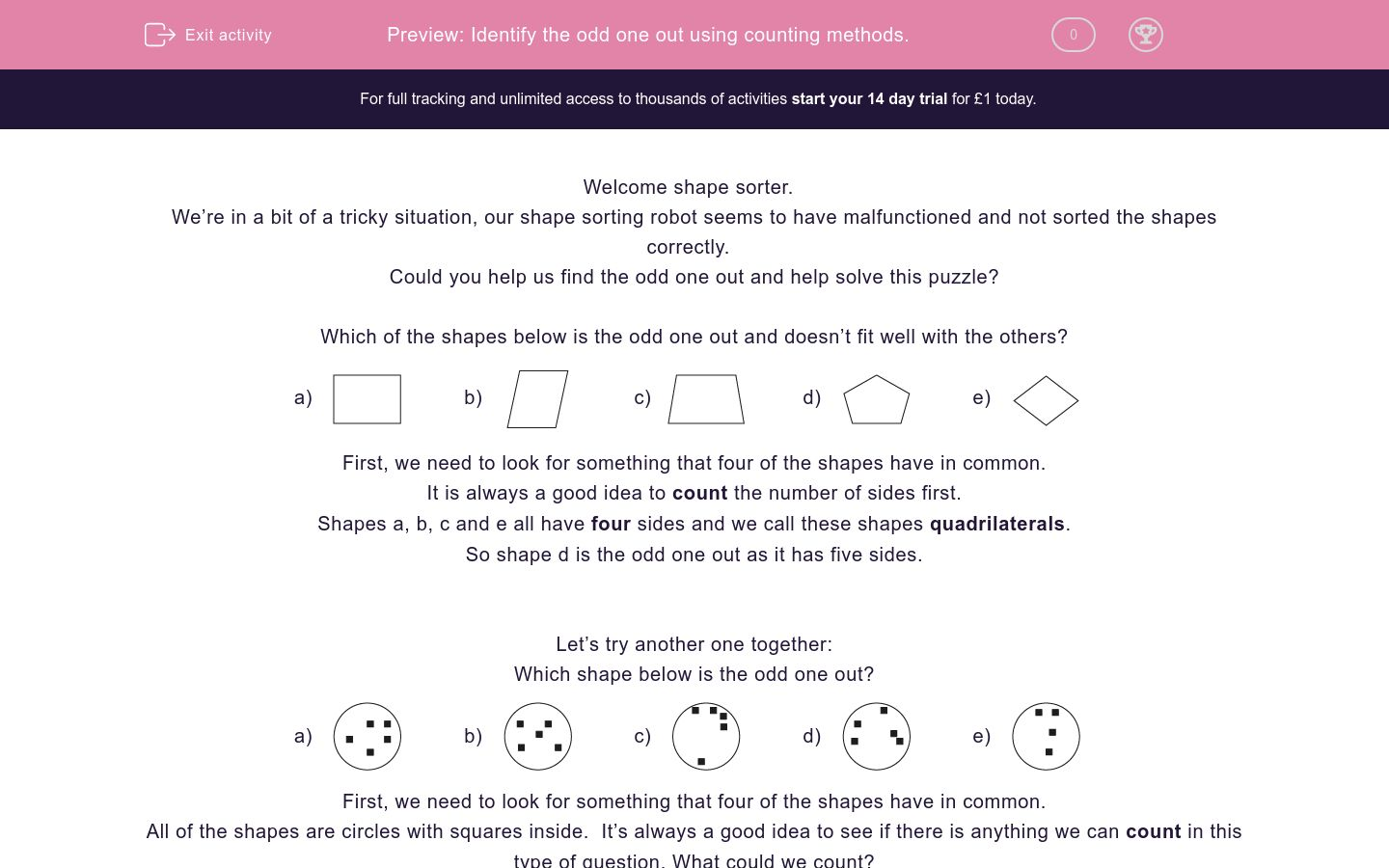Key stage:  KS 2

Curriculum topic:   Spatial and Non-Verbal Reasoning

Curriculum subtopic:   Odd One Out

Difficulty level:### QUESTION 1 of 10

Welcome shape sorter.

We’re in a bit of a tricky situation, our shape sorting robot seems to have malfunctioned and not sorted the shapes correctly.

Could you help us find the odd one out and help solve this puzzle?

Which of the shapes below is the odd one out and doesn’t fit well with the others?

a)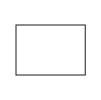b)c)d)e)First, we need to look for something that four of the shapes have in common.

It is always a good idea to count the number of sides first.

Shapes a, b, c and e all have four sides and we call these shapes quadrilaterals.

So shape d is the odd one out as it has five sides.

Let’s try another one together:

Which shape below is the odd one out?

a)b)c)d)e)First, we need to look for something that four of the shapes have in common.

All of the shapes are circles with squares inside.  It’s always a good idea to see if there is anything we can count in this type of question. What could we count?

If you count the number of squares, you will notice that shapes a, b, c and d all have five squares inside them.

This means that shape e is the odd one out as it has only four squares in the middle not five.

It’s now your turn to identify the odd shape.

The theme is counting so always be on the lookout for what you can count.

Good luck shape sorter!

Which of the shapes below is the odd one out and doesn't fit well with the others?

a)b)c)d)e)Which of the shapes below is the odd one out and doesn't fit well with the others?

a)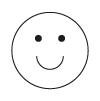b)c)d)e)Which of the shapes below is the odd one out and doesn't fit well with the others?

a)b)c)d)e)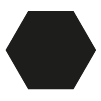Which of the shapes below is the odd one out and doesn't fit well with the others?

a)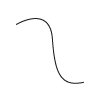b)c)d)e)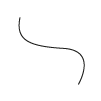Which of the shapes below is the odd one out and doesn't fit well with the others?

a)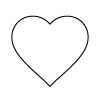b)c)d)e)Which of the shapes below is the odd one out and doesn't fit well with the others?

a)b)c)d)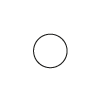e)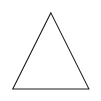Which of the shapes below is the odd one out and doesn't fit well with the others?

a)b)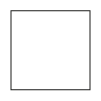c)d)e)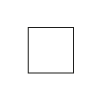Which of the shapes below is the odd one out and doesn't fit well with the others?

a)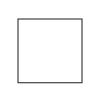b)c)d)e)Which of the shapes below is the odd one out and doesn't fit well with the others?

a)b)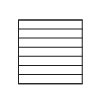c)d)e)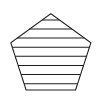Which of the shapes below is the odd one out and doesn't fit well with the others?

a)b)c)d)e)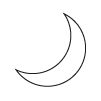• Question 1

Which of the shapes below is the odd one out and doesn't fit well with the others?

c)EDDIE SAYS
Let's look for what the shapes have in common first. If we look carefully, we can see that four of the five shapes are triangles and one is a square. The square doesn't fit in with the triangles so option c is the odd one out. Did you know that a square is also a type of quadrilateral as it has four sides?
• Question 2

Which of the shapes below is the odd one out and doesn't fit well with the others?

d)EDDIE SAYS
Let's look for what the shapes have in common first. Did you notice that four of the five shapes are happy and one is sad? So option d is the odd one out as it has a sad rather than a smiley face. Option d is called a trapezium - it is a sad trapezium that we need to cheer up!
• Question 3

Which of the shapes below is the odd one out and doesn't fit well with the others?

b)EDDIE SAYS
At first glance, all of the shapes look totally different here! One is a rectangle, one is a circle, one is a square, one is a triangle and one is a hexagon. What do they all have in common? You may notice that four of the shapes are shaded and one is not. This makes option b (the blank shape) the odd one out, as it is the only one which isn't shaded. Did you spot that sneaky shading top detective?
• Question 4

Which of the shapes below is the odd one out and doesn't fit well with the others?

d)EDDIE SAYS
Let's look for what these images have in common first. Four of the lines are wiggly and one is straight. This means that option d is the odd one out as it isn't wiggly. All of the lines are facing different directions here, so we know this doesn't matter here. Be careful to avoid red herrings like this!
• Question 5

Which of the shapes below is the odd one out and doesn't fit well with the others?

b)EDDIE SAYS
Let's look for what these images have in common first. This time four of the five shapes are love hearts. So option b must be the odd one out, as it is not a love heart. Now I LOVED that question!
• Question 6

Which of the shapes below is the odd one out and doesn't fit well with the others?

e)EDDIE SAYS
Let's look for what these images have in common first. Here the first four images are round and the last image isn't. Do you know what type of shape this is? Option e must be the odd one out as it is the only which isn't round. Let's get ROUND to the next question!
• Question 7

Which of the shapes below is the odd one out and doesn't fit well with the others?

c)EDDIE SAYS
This one is a little tricky as your eyes can be confused by the different sizes and shapes at the same time. If you look carefully you can see that four of the images are squares. The sneaky squares are trying to catch us out as they are all different sizes, but that doesn't matter! Option c is still the odd one out as it is the only shape which isn't a square. Don't get SQUARE eyes looking at all these shapes!
• Question 8

Which of the shapes below is the odd one out and doesn't fit well with the others?

c)EDDIE SAYS
Again, this is a little confusing as there are lots of different shapes here. If we look carefully we can see that options a, b, d and e have four sides even though one is a square and three are rectangles. So option c is the odd one out as it has six sides not four. Did you know that four-sided shapes are called quadrilaterals?
• Question 9

Which of the shapes below is the odd one out and doesn't fit well with the others?

a)EDDIE SAYS
This time the pattern inside the shape is really important. Options b, c, d and e are all stripey but option a is not. Sadly the square is not looking as snazzy as his shape friends! This means that option a is the odd one out as it isn't stripey.
• Question 10

Which of the shapes below is the odd one out and doesn't fit well with the others?

c)EDDIE SAYS
Here we have four crescent moons and one random rectangle. This means that option c is the odd one out, as it isn't a crescent moon. I hope that looking at these MOONS hasn't made you sleepy! That was the final question so you can have a rest now - well done!
---- OR ----